# How to Find Outliers in Excel?

• Last Updated : 28 Mar, 2022

Outliers as the name suggest are something that doesn’t fall in the required/given range. Outliers in statistics need to be removed because they affect the decision that is to be made after performing the required calculations. Outliers generally make the decision skewed i.e they move the decision in a positive or negative direction. Sometimes it is easy to find an outlier by looking at the data but it is difficult to find an outlier when the data is large. We’ll see this with the help of an example, given a dataset and you need to perform the average of the dataset 1, 89, 57, 100, 150, 139, 49, 87, 200, 250. So, the average of the given data set is 112.2. But, it is clearly visible that 1, 200, and 250 are ranges that are too small or too large to be a part of the dataset. These ranges are known as outliers in data. After removing the outliers, the average becomes 95.85. It is evidently seen from the above example that an outlier will make decisions based.

### Finding Outliers using Sorting in Excel

This is one of the easiest ways to find outliers in MS excel when your data is not huge because by having a look at the data you’ll get to know about the values that are far away from the originally recorded values.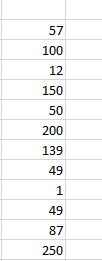From the above image, we can clearly tell that the data is not sorted and hence it would take some time for us to identify outliers.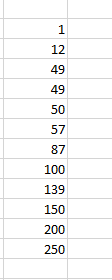While looking at Img. 2, we can clearly say that the numbers 1, 200, and 250 are outliers.

### Finding Outliers using LARGE/SMALL Excel Function

Another way to find outlier is by using built-in MS Excel functions known as LARGE and SMALL. The LARGE function will return the largest value from the array of data and the SMALL function will return the smallest value. Here, we will be using a LARGE and SMALL function which is an in-built function in Microsoft excel. Consider the example used above: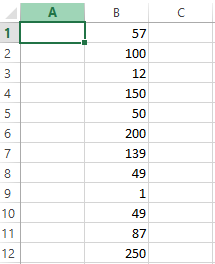LARGE Function Syntax:

LARGE(\$B\$1:\$B\$12, 1)

Here, we are passing an array and a number. The array has the dataset for which we have to find the outlier and the number, 1, represents the first largest number from the array. If we use 2, it will return the second largest value from the array. Now when we use this function in the above example, we will get the following output: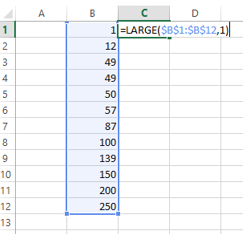SMALL Function Syntax:

SMALL(\$B\$1:\$B\$12, 1)

The syntax and pass-on value are the same. Now when we use this function in the above example, we will get the following output: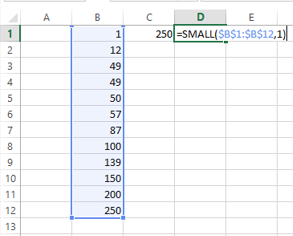Note: If there are multiple outliers in the data then you have to use the function again and again.

### Finding Outliers using Inter Quartile Range(IQR)

The data presented in the above example has a small sample size but when it comes to a real-life situation, the data can be huge, and that’s where the original problem arrives. As per IQR, An outlier is any point of data that lies over 1.5 times IQRs below the first quartile (Q1) and 1.5 times IQR above the third quartile (Q3)in a data set.

Formula is

High = Q3 + 1.5 * IQR

Low = Q1 – 1.5 * IQR

Finding Outliers using the following steps:

Step 1: Open the worksheet where the data to find outlier is stored.

Step 2: Add the function QUARTILE(array, quart), where an array is the data set for which the quartile is being calculated and a quart is the quartile number. In our case, the quart is 1 because we wish to calculate the 1st quartile to calculate the lowest outlier.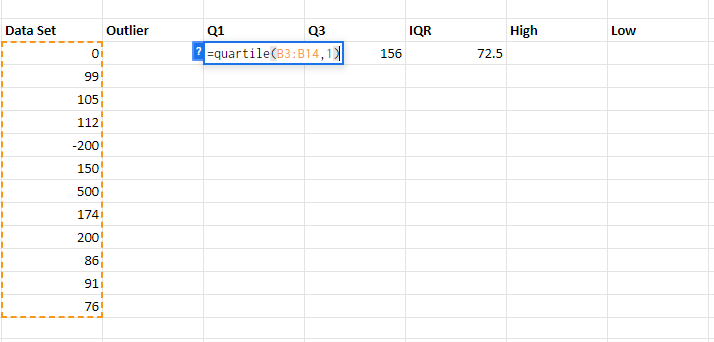Step 3: Similar to step 2 add the quartile formula under Q3 and write 3 as quart number because we wish to calculate the 3rd quartile i.e 75th percentile to calculate the highest quartile value.

Step 4: Inter Quartile Range or IQR is Q3-Q1, put the formula to get the IQR value.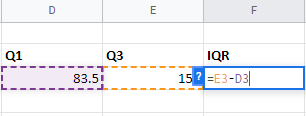Step 5: To find the High value, the formula is Q3+(1.5*IQR). Similarly, for Low value, the formula is Q1-(1.5*IQR)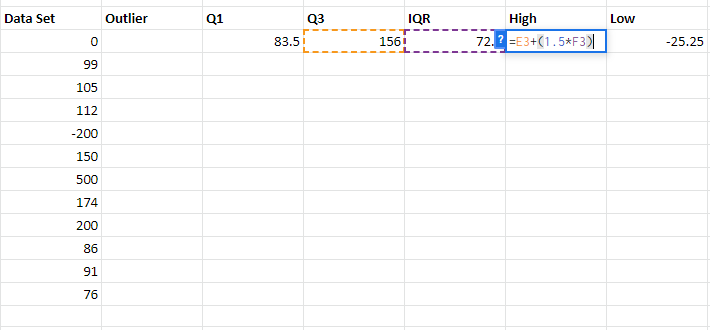Step 6: To find whether the number in the data set is an outlier or not, we need to check whether the data entry is higher than the High value or lower than the Low value. To perform this we will use the OR function. The formula will be OR(B3>\$G\$3, B3<\$H\$3). Put the formula in the required cell and drag down the cell adjacent to the last data set, if the value returns TRUE, then the data is an outlier otherwise not.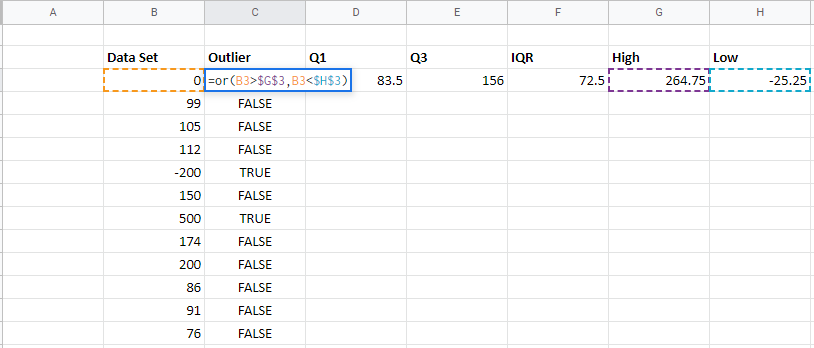Since you’ve checked for the outlier data. Now you can remove the outliers and use the rest data for calculations and get unbiased results.

My Personal Notes arrow_drop_up
Recommended Articles
Page :